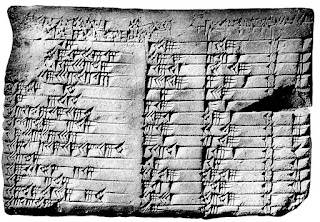## Tuesday, 17 November 2020

### Constants and calculationsBabylonian equations
Carroll uses natural units in his book as do I on this web site. In natural units the speed of light, Planck's constant and Boltzmann's constants $c=\hbar=h/2\pi=k=1$. In our equations these terms are left out. They need to be put in again to do real calculations and it's not always obvious how to do it. When Carroll does calculations he often uses centimeters and grams. I prefer to use SI units: meters and kilograms.

It is also common to use geometric units which are units in which $c=G=1$ (so mass and energy have the same units as length and time). You cannot also have $\hbar=1$ in these units.

In natural units the Schwarzschild radius is $R_S=2GM$. I suppose that in geometric units it is $R_S=2M$. In SI units it is $2GM/c^2$. The $c^2$ makes a big difference and is easy to forget!

The document contains a list in SI units of the values of universal constants and other handy constants like the mass of the sun. The table also gives the conversion factors from natural to SI units, which are derived from their dimensions. Two examples of their use are given at the end followed by a list of small and large prefixes (Terra, peta, pico etc) and their meanings.